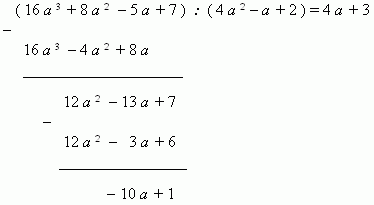# Division of polynomials

Division of polynomials (quotient, remainder). Long division.

Division of polynomials. What means to divide one polynomial P by another Q ? It means to find polynomials M ( quotient ) and N ( remainder ), satisfying the two requirements:

1).  An equality MQ + N = P takes place;
2).  A degree of polynomial N is less than a degree of polynomial Q .

Division of polynomials can be done by the following scheme ( long division ):1)  Divide the first term 16 a 3 of the dividend by the first term  4 a 2 of the divisor;  the result  4 a is the first term of the quotient.

2)  Multiply the received term 4 a by the divisor 4 a 2 – a + 2; write the result 16 a 3 4 a 2 + 8 a under the dividend, one similar term under another.

3)  Subtract terms of the result from the corresponding terms of the dividend and move down the next by the order term 7 of the dividend; the remainder is 12 a 2 13 a + 7 .

4)  Divide the first term 12 a 2 of this expression by the first term 4 a 2 of the divisor;  the result 3 is the second term of the quotient.

5)  Multiply the received second term 3 by the divisor 4 a 2 – a + 2; write the result 12 a 2 3 a + 6 again under the dividend, one similar term under another.

6)  Subtract terms of the result from the corresponding terms of the previous remainder and receive the second remainder:
10 a + 1. Its degree is less than the divisor degree,  therefore the division has been finished. The quotient is  4 a + 3,
the remainder is
10 a + 1.